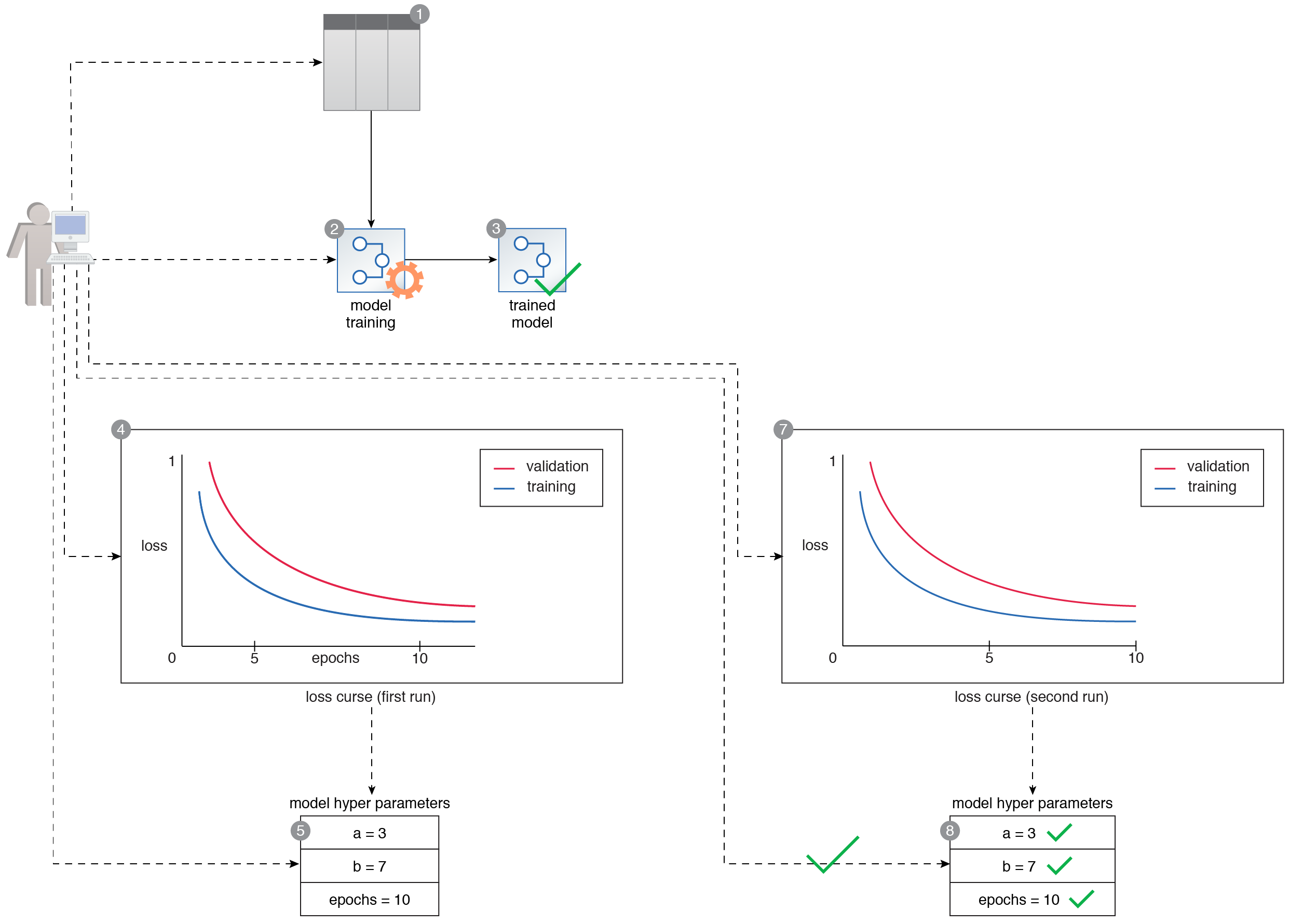# Training Performance Evaluation

Artificial Intelligence (AI) Patterns, Neurons and Neural Networks > Model Evaluation Patterns > Training Performance Evaluation

## Training Performance Evaluation

How can confidence be established in the efficacy of a neural network model at training time?

### Problem

Productionalizing a trained neural network model that makes incorrect predictions can seriously jeopardize the effectiveness and reliability of a system.

OR

Different models can be developed to solve a particular category of deep learning problem. However, not knowing which model is most suitable may lead to choosing a not-so-optimum model for the production system with the further consequence of sub-par system performance.

### Solution

The model’s performance is quantified via established model evaluation techniques that make it possible to estimate the performance of a single model or to compare different models.

### Application

Based on the type of deep learning problem (supervised or unsupervised) various statistics and visualizations are generated. For regression problems, the loss metric is used. For classification problems, both accuracy and loss metrics are can be used. A confusion matrix may also be used.A data scientist prepares a training dataset (1). The training dataset is then used to train a multi-class neural network classifier (2, 3). The data scientist generates the error metric for each epoch and plots it as a line chart. They observe that the validation error first decreases but then starts to increase, suggesting that the model is overfitting (4). Based on the result, they retune the epoch parameter value and then retrain the model again for a second training run (5, 6). The metrics are evaluated again for the second training run. The error curve suggests that the model is not suffering from overfitting anymore (7). After training the model twice, the data scientist is satisfied with the performance of the model and selects the parameters used in the second training run as the final values of the model’s hyperparameters (8).